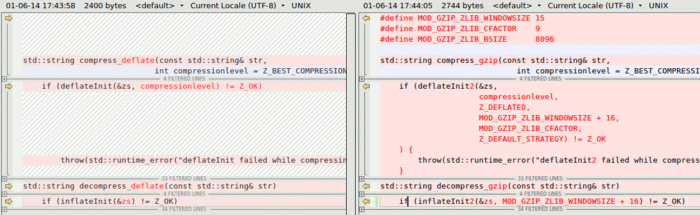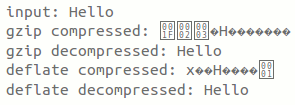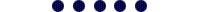Neither one nor Many

June 1 2014

## Deflate and Gzip compress and decompress functions

When implementing a gzip compression & decompression for my blog. I stumbled upon two C++ functions by Timo Bingmann:

• string : compress_string(string)
• string : decompress_string(string)

Renamed these and created two gzip versions from them:

• string : compress_deflate(string)
• string : decompress_deflate(string)
• string : compress_gzip(string)
• string : decompress_gzip(string)

These are the differences:## Complete source

```#include <string>
#include <sstream>
#include <stdexcept>

#include <string.h>
#include "zlib.h"

using std::string;
using std::stringstream;

// Found these here http://mail-archives.apache.org/mod_mbox/trafficserver-dev/201110.mbox/%3CCACJPjhYf=+br1W39vyazP=ix
//eQZ-4Gh9-U6TtiEdReG3S4ZZng@mail.gmail.com%3E
#define MOD_GZIP_ZLIB_WINDOWSIZE 15
#define MOD_GZIP_ZLIB_CFACTOR    9
#define MOD_GZIP_ZLIB_BSIZE      8096

// Found this one here: http://panthema.net/2007/0328-ZLibString.html, author is Timo Bingmann
// edited version
/** Compress a STL string using zlib with given compression level and return
* the binary data. */
std::string compress_gzip(const std::string& str,
int compressionlevel = Z_BEST_COMPRESSION)
{
z_stream zs;                        // z_stream is zlib's control structure
memset(&zs, 0, sizeof(zs));

if (deflateInit2(&zs,
compressionlevel,
Z_DEFLATED,
MOD_GZIP_ZLIB_WINDOWSIZE + 16,
MOD_GZIP_ZLIB_CFACTOR,
Z_DEFAULT_STRATEGY) != Z_OK
) {
throw(std::runtime_error("deflateInit2 failed while compressing."));
}

zs.next_in = (Bytef*)str.data();
zs.avail_in = str.size();           // set the z_stream's input

int ret;
char outbuffer;
std::string outstring;

// retrieve the compressed bytes blockwise
do {
zs.next_out = reinterpret_cast<Bytef*>(outbuffer);
zs.avail_out = sizeof(outbuffer);

ret = deflate(&zs, Z_FINISH);

if (outstring.size() < zs.total_out) {
// append the block to the output string
outstring.append(outbuffer,
zs.total_out - outstring.size());
}
} while (ret == Z_OK);

deflateEnd(&zs);

if (ret != Z_STREAM_END) {          // an error occurred that was not EOF
std::ostringstream oss;
oss << "Exception during zlib compression: (" << ret << ") " << zs.msg;
throw(std::runtime_error(oss.str()));
}

return outstring;
}

// Found this one here: http://panthema.net/2007/0328-ZLibString.html, author is Timo Bingmann
/** Compress a STL string using zlib with given compression level and return
* the binary data. */
std::string compress_deflate(const std::string& str,
int compressionlevel = Z_BEST_COMPRESSION)
{
z_stream zs;                        // z_stream is zlib's control structure
memset(&zs, 0, sizeof(zs));

if (deflateInit(&zs, compressionlevel) != Z_OK)
throw(std::runtime_error("deflateInit failed while compressing."));

zs.next_in = (Bytef*)str.data();
zs.avail_in = str.size();           // set the z_stream's input

int ret;
char outbuffer;
std::string outstring;

// retrieve the compressed bytes blockwise
do {
zs.next_out = reinterpret_cast<Bytef*>(outbuffer);
zs.avail_out = sizeof(outbuffer);

ret = deflate(&zs, Z_FINISH);

if (outstring.size() < zs.total_out) {
// append the block to the output string
outstring.append(outbuffer,
zs.total_out - outstring.size());
}
} while (ret == Z_OK);

deflateEnd(&zs);

if (ret != Z_STREAM_END) {          // an error occurred that was not EOF
std::ostringstream oss;
oss << "Exception during zlib compression: (" << ret << ") " << zs.msg;
throw(std::runtime_error(oss.str()));
}

return outstring;
}

/** Decompress an STL string using zlib and return the original data. */
std::string decompress_deflate(const std::string& str)
{
z_stream zs;                        // z_stream is zlib's control structure
memset(&zs, 0, sizeof(zs));

if (inflateInit(&zs) != Z_OK)
throw(std::runtime_error("inflateInit failed while decompressing."));

zs.next_in = (Bytef*)str.data();
zs.avail_in = str.size();

int ret;
char outbuffer;
std::string outstring;

// get the decompressed bytes blockwise using repeated calls to inflate
do {
zs.next_out = reinterpret_cast<Bytef*>(outbuffer);
zs.avail_out = sizeof(outbuffer);

ret = inflate(&zs, 0);

if (outstring.size() < zs.total_out) {
outstring.append(outbuffer,
zs.total_out - outstring.size());
}

} while (ret == Z_OK);

inflateEnd(&zs);

if (ret != Z_STREAM_END) {          // an error occurred that was not EOF
std::ostringstream oss;
oss << "Exception during zlib decompression: (" << ret << ") "
<< zs.msg;
throw(std::runtime_error(oss.str()));
}

return outstring;
}

std::string decompress_gzip(const std::string& str)
{
z_stream zs;                        // z_stream is zlib's control structure
memset(&zs, 0, sizeof(zs));

if (inflateInit2(&zs, MOD_GZIP_ZLIB_WINDOWSIZE + 16) != Z_OK)
throw(std::runtime_error("inflateInit failed while decompressing."));

zs.next_in = (Bytef*)str.data();
zs.avail_in = str.size();

int ret;
char outbuffer;
std::string outstring;

// get the decompressed bytes blockwise using repeated calls to inflate
do {
zs.next_out = reinterpret_cast<Bytef*>(outbuffer);
zs.avail_out = sizeof(outbuffer);

ret = inflate(&zs, 0);

if (outstring.size() < zs.total_out) {
outstring.append(outbuffer,
zs.total_out - outstring.size());
}

} while (ret == Z_OK);

inflateEnd(&zs);

if (ret != Z_STREAM_END) {          // an error occurred that was not EOF
std::ostringstream oss;
oss << "Exception during zlib decompression: (" << ret << ") "
<< zs.msg;
throw(std::runtime_error(oss.str()));
}

return outstring;
}

// Compile: g++ -std=c++11 % -lz
// Run: ./a.out

#include <iostream>

int main()
{
std::string s = "Hello";

std::cout << "input: " << s << std::endl;
std::cout << "gzip compressed: " << compress_gzip(s) << std::endl;;
std::cout << "gzip decompressed: " << decompress_gzip(compress_gzip(s)) << std::endl;;
std::cout << "deflate compressed: " << compress_deflate(s) << std::endl;;
std::cout << "deflate decompressed: " << decompress_deflate(compress_deflate(s)) << std::endl;;
}

```

## Compile

`g++ -std=c++11 % -lz`

## Run

`./a.out`

## OutputThanks. I'd seen this but hadn't clued in enough with the differences between compress/gzip so choosing the right options and using deflateInit2 has done what I needed. I was compressing instead of gzipping.Thank you so much for this simple sample.
I've been stumble into so many places until I found what I needed here.

This is the functions I used: decompress_gzip
But I can tell the rest of them work like a charm.

Thank you and congratulations for such a simple sample to use this functions.Comment may not be visible immediately, because I process everything manually.**

**) I plan to automate this.., but it's on my ToDo since for ever..

Author:Ray Burgemeestre
february 23th, 1984

Topics:
C++, Linux, Webdev

Other interests:
Music, Art, Zen